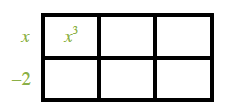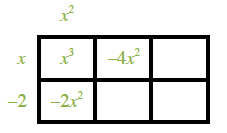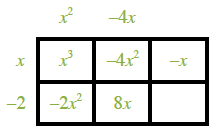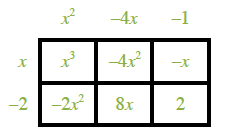### Home > INT3 > Chapter 8 > Lesson 8.3.2 > Problem8-121

8-121.

Given the polynomial $p\left(x\right) = x^{3} – 6x^{2} + 7x + 2$:

1. Use the Remainder Theorem to determine $p\left(2\right)$.

Divide the polynomial by $\left(x − 2\right)$. What is the remainder?

2. Now use the Factor Theorem to determine one factor of $x^{3} – 6x^{2} + 7x + 2$. (See the Math Notes box in this lesson.)

Since $p\left(2\right) = 0, x = 2$ is the zero of the function. What is the corresponding factor?

$\left(x − 2\right)$

Try using an area model. A 2 by 3 rectangle, labeled as follows: left edge, x, minus 2, interior top, left, x, cubed.

Labels added: top edge, left, x, squared, interior bottom, left, negative 2, x, squared, interior top, middle, negative 4, x squared.

Labels added: top edge, middle, negative 4, x, interior bottom, middle, 8, x, interior top, right, negative x.

Labels added: top edge, right, negative 1, interior bottom, right, 2.

$\left(x^{2} − 4x − 1\right)$4. What are all the solutions of $x^{3} – 6x^{2} + 7x + 2 = 0$?

See part (a) for one solution.

Use the Quadratic Formula to find the solutions to
$0 = x^{2} − 4x − 1$.

$x = 2, 2 \pm \sqrt{5}$

$x=\frac{4 \pm \sqrt{16 + 4}}{2}$